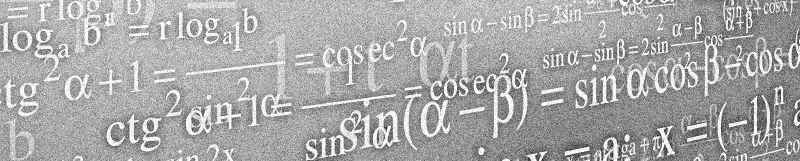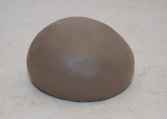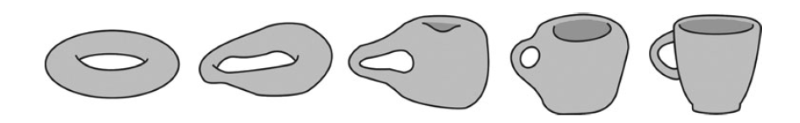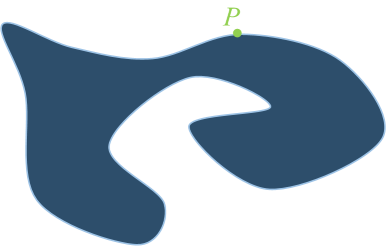﻿ The Poincaré conjecture# Mathematics

I’m not a mathematician. I just pretend. There are some real mathematicians in the World, and they work on some fabulously complicated problems. Still, however, there are many classic problems in mathematics that have never been solved.At the turn of the Century, the Clay Mathematics Institute created a list of seven particularly challenging problems they’d like to see solved. This collection was given the title The Millennium Problems and, to incentivize research, a cash prize of \$1,000,000 was offered to the solver of any of these problems.Fifteen years later, just one of these problems has been solved, and this is the Poincaré conjecture. This was solved by the Russian mathematician Grigori Perelman. Perelman was offered the one million dollars cash prize, and also awarded the Field’s Medal (The ‘Nobel Prize for mathematics’) for his work, but declined both of them stating: “I'm not interested in money or fame; I don't want to be on display like an animal in a zoo.”

“I'm not interested in money or fame; I don't want to be on display like an animal in a zoo.”*

 * The \$1 million that was declined by Perelman is being put to good use: the Clay Foundation prize money is being used to endow the Poincaré Chair. Launched in January 2013 by the Institut Henri Poincaré and the Clay Mathematics Institute, this chair offers exceptional young mathematicians ideal working conditions to develop their scientific projects.# Poincaré conjecture

This problem was originally conjectured by Henri Poincaré, and concerns a space that locally looks like ordinary three-dimensional space but is connected, finite in size, and lacks any boundary. Huh?

I can’t claim to even begin to understand much of Perelman’s paper, but it seems his proof leveraged some earlier research into the problem using something called Ricci Flow. Ricci flow looked pretty interesting, and being a nerd, it seemed like a fun topic to write some code around (but just in 2D for me!) More in this later, but first a little more background:

### Topology

There are many branches of mathematics. Most of us are familiar with geometry, which is the study of shapes and their properties (and is arguably, probably the oldest form of mathematics. People studied shapes long before the concept of numbers). In geometry, typically, things remain fixed, and we study the relationships between shape, size, dimensions and relative positions of objects.

 Another branch of mathematics is topology, and this is the study of the properties of objects and spaces that are preserved under deformations such as stretching, bending and shearing (but not tearing, bonding or welding). The connectedness and compactness of shapes is important in topology, but not their areas, volumes or relative positions. A useful analogy (in two dimensions), is an infinitely stretchy rubber sheet. If you draw something on a rubber sheet, then stretch, bend and distort the sheet, no matter what you do to the sheet (as long as you don’t make a hole in it, or bond it to itself), the before and after meshes are topologically equivalent.### Mind The GapImage: Tom Page One of the common examples of the usefulness of topological equivalency is a subway map. When we look at a subway map, we’re interested in which line(s) to take to get to our destination, how many stops we have to pass, and where we might need to change trains. A London Underground map is shown below on the left. The stations are shown in their correct order, but the position of the stations has been moved to make the map easier to read. On the right is a map showing the actual physical locations of the stations (but even this is not geometrically accurate because, even though the stations are in the correct geographic coordinates, the path of the tracks connecting the stations is not following the subtle curves of the actual physical location of the track).

The two maps are, however, both topologically equivalent. They represent the same connectedness. Stations appear in the correct order (even if they are rendered at locations and at separations non-representative of real life). The junctions (which lines cross and interchange) are the same, and no station has moved from inside one bounding collection of lines to a different bounded region. No breaks have occurred in the lines, and no station has a different number of lines serving it from before.If you printed a scale map of the London onto a sheet of rubber, and draw on the exact path of the subway lines, with patience, you could stretch the rubber to make a map that looks like the tube map seen above.

The two maps are said to be homeomorphic

### Three Dimensions

If we move into three dimensions, things get a little more complicated. Instead of imagining sheets of rubber, mathematicians imagine infinitely stretchy blobs of clay. If you start with a solid shape (like a spherical blob of clay), it’s possible to distort this to make another solid shape, like a cube, or a pancake, or cigar shape. All these shapes (or manifolds as mathematicians call them), are topologically equivalent.If you start with a ball of clay you can mold it into a cube. As long as you don’t puncture it (to make a hole), or bend it and attempt to join it back on itself (either making another hole, or closing one off), then any shape you can create is topologically equivalent.(Cutting is not allowed either!)A doughnut shape (torus), however, cannot be made from a ball. You can’t make the hole required without puncturing through the surface of the clay. No matter how much you try; you can’t distort a ball of clay to make a ring.

You can, however, turn a cup into a doughnut (there is a hole by the handle), and stretching and pulling can make this the center of a toroid. This is the basis for a geeky joke that a mathematician does not know the difference between the two.A topologist is a mathematician who doesn’t know the difference between a doughnut and a coffee cup!

 Other real world examples of a branch of topology are circuit diagrams and wiring diagrams. If you make an electric circuit it does not matter the path you route the wires between connections. As long as the correct components are connected in the correct way, the circuit will work (With wiring, things are less strict. You can wind and wrap cables into knots; both with themselves and other wires, and as long as the components to be connected are the right ones, and no others, the circuits will be equivalent).### Back to Poincaré

If you put a loop of string around a sphere, and tighten it, then the loop gets smaller and smaller, and eventually reduces to a point. This always happens, and we call this kind of shape having a simply connected surface. However, if you wrap a string around a doughnut, and pull, it does not tighten to a point. This is because the doughnut has a hole.Image: Salix alba

What Poincaré postulated is that if you took any shape (that has no holes), and it is bounded (does not go on forever), then you could squash it into a sphere. (Meaning that the sphere is the simplest form of the manifold in three dimensions). I know this might sound kinda simple, but proving this to be true is what Perleman received the prize for. Interestingly, this conjecture had been proved earlier for hyper-spheres of more dimensions than three, but not for three.

To prove the conjecture, Perelman used something called Ricci Flow. Let's take a look at something a little simpler called curve shortening to get us moving in the right direction.

### Curve ShorteningLet's get back to two dimensions. If we take a closed curve, it's possible to deform this shape by moving each point on the edge according to some rules. We're going to move each point a distance (inversely) proportional to the local radius of curvature of the shape at that point. For every point, we find the tangent, and the local curvature. If the radius of the curvature is small (tight curve), we shall displace the point a large distance perpendicular to the tangent. If the radius of curvature is large (very gentle curve), we will displace that point only a small amount. The displacements are made towards the center of the curvature circle.

If the curvature is convex, this means we displace the point further inside the shape (towards the center of the curvature circle):If the curvature at a point is concave, the point will be displaced outwards:The tighter the curve, the larger the displacement. We perform this displacement operation for every point of the curve, and when finished we will have generated a slightly different shape.

We then repeat this 'flow' calculation again and again …

### Interesting Results

If we perform this operation over and over again, something interesting happens. First of all, the curve gets shorter with each iteration, but more interestingly, over time, no matter how complicated a shape we start off with, the shape turns circular.

Then, when it is pretty much circular in shape, it starts to collapse on itself. This is not surprising; once a circle like shape begins to form, all displacements point towards a central point (If the shape is not quite circular yet, but still convex, the more 'pointed' parts of the shape will get displaced in faster than the 'straighter' edges and so this makes the shape more circular).

Eventually, the circle gets so small that the radius become infinitesimally small, and the point turns into a singularity. Below is an example:If you want to see some more examples, look here.

Even when the curve is complex and overlaps itself, the curve shortening works (as you can see in the examples shown in the link above). As a curve unwinds it has a hypnotic little snapping action when the loops are collapsed and it gives the operation an, almost, organic feel.

# Try it yourself

Below is an applet that performs curve shortening. It's pre-loaded with a complicated shape. You can start and stop the operation using the button below the grid. After watching the pre-loaded shape you can draw your own custom shape on the grid and run that.

I have to admit it's pretty fun to play with!

Also fascinating is that if you draw a curve that is simple (does not overlap itself), then throughout the entire shrinking operation, the curve never crosses over itself. This is given the name Gage-Hamilton-Grayson Theorem. (The last example on this page shows a spiral shape shrinking down).

Another interesting application of this theorem is the rendezvous problem for a collection of autonomous vehicles. Imagine you have a collection or robots (at arbitrary positions) and you want them all to rendezvous at a common point. Nice!

### Back to 3D

Our curve shortening works fine in 2D, but when we graduate to 3D, we can't just use the radius of curvature and a tangent, and instead we need to use a tangential plane touching the surface at one point and calculate the mean curvature in 3D space.

More complicated things can happen in 3D, and progress can get stuck. Imagine, for instance, a dumb-bell shape, with two spherical lobes on each side, connected by a thin straight section. The thin straight section has, essentially an infinite radius, and thus does not get moved.

As I understand it (and this is being generous on my part), the key to Perelman's solution is the identification of these problem regions so that they can be quarantined and then 'surgery' applied to these weird regions to allow progress to continue.

You can find a complete list of all the articles here.Click here to receive email alerts on new articles.